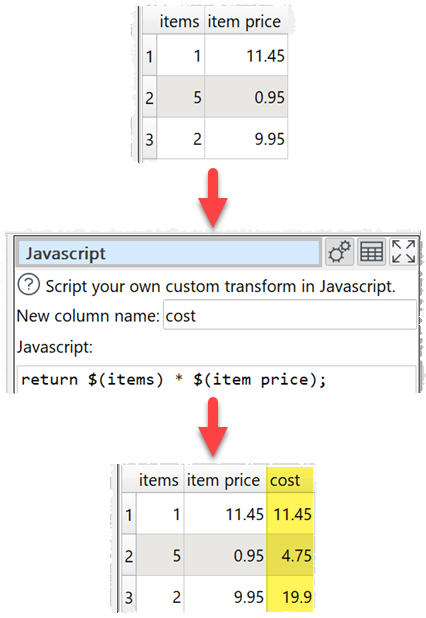﻿ Reference > Transforms > Javascript

# JavaScript

## Description

Create a custom transform using JavaScript (ECMAScript).

Easy Data Transform allows you to carry out a wide range of data transformations without programming. But sometimes you might need a specialist transformation that can't be done with the built-in transforms. For that you can use the JavaScript transform. It allows you to write the body of a JavaScript function, to calculate a value for each row in a new column. Existing column values can be used as variables.

JavaScript is a fully-fledged programming language and can perform arbitrarily complex transforms. It can handle numbers, dates and text.

## Examples

Multiple the value in column 'items' by the value in column 'item price':To concatenate 'last' and 'first' columns with a comma and a space:

return \$(last) + ', ' + \$(first);

To calculate the biggest of columns 'v1' and 'v2':

return Math.max( \$(v1), \$(v2) );

To determine whether phone numbers in the 'phone_num' column are valid using a regular expression:

const validPhoneNum = /^[\+]?[(]?[0-9]{3}[)]?[-\s\.]?[0-9]{3}[-\s\.]?[0-9]{4,6}\$/;

if ( validPhoneNum.test( \$(phone_num) ) )

return "valid";

else

return "invalid";

To replace the last comma in the 'Location' column with a ';' using a regular expression:

return \$(Location).replace(/(.*),([^ ]*)\$/, '\$1;\$2');

To calculate the number of years difference between JavaScript compatible dates in column 1 and column 2:

return new Date( \$(1) ).getFullYear() - new Date( \$(2) ).getFullYear();

To calculate the number of milliseconds between a datetime in the 'datetime' column and 1st Jan 2000:

return new Date( \$(date) ) - new Date( "2000-01-01T00:00" );

To calculate the number of whole days difference between a date in the 'created' column and today (negative for future dates):

return Math.floor( ( new Date() - new Date( \$(created) ) ) / ( 1000*60*60*24 ) );

To use the value of the 'n' column if it is a number and 0 if it isn't:

if ( isNaN( \$(n) ) )

return 0;

else

return \$(n);

To reverse the text in the 'key' column:

var a = \$(key);

var newString = "";

for (var i = a.length - 1; i >= 0; i--) {

newString += a[i];

}

return newString;

To convert meters to feet using a function:

function metersToFeet( x )

{

return 3.28084 * x;

}

return metersToFeet( \$(meters) );

One.

## Options

Enter your script into the JavaScript field. The script should be the body of a JavaScript function.

Select a column from Insert variable to add that column variable into the JavaScript field at the current cursor position.

Click the Evaluate button to evaluate your JavaScript expression over every row and show any errors.

## Notes

The JavaScript transform is calculated every time:

oThe Evaluate button is pressed.

oThe JavaScript transform item is unselected in the Center pane and script changes have been made without the Evaluate button being clicked.

oAn item upstream of it changes.

A numeric value  is passed as a JavaScript number variable. true and false are passed as a JavaScript boolean variable. Everything else is passed as a JavaScript string variable.

An empty cell is passed as an empty JavaScript string variable (""), not null. To test if the "v" column has a blank or empty value:

if ( \$(v).length === 0 )

{

// empty cell

}

else if ( String( \$(v) ).trim().length === 0 )

{

// blank cell

}

Numeric values should use dot ('.') as the decimal separator and have no group separator. E.g. 1234.5 is valid, but 1,234.5 and 1.234,5 are not, regardless of the locale. You can use the Num Format and Replace transforms to put numeric data in the correct format before processing a JavaScript transform.

The new column is added at the right end. You can change the column order with Reorder Cols and the column name with Rename Cols.

The JavaScript Date() object evaluates to the number of milliseconds since 1 January 1970 UTC.

Date values passed to JavaScript Date() objects should be in ISO ('yyyy-mm-dd') format, e.g. '2020-01-31' (not '2020-1-31').

If you want to carry out your transform across more than one dataset, you should merge them first, e.g. using Join.

The JavaScript transform is very versatile and quite fast. But is not as fast as built-in transforms. So we recommend you use built-in transforms where possible.

JavaScript running in Easy Data Transform is not 'sandboxed' and has the same privileges as the Easy Data Transform executable. However the JavaScript does not have access to window(), XMLHttpRequest() or ActiveXObject(). So we aren't aware of any way that a bad actor could damage your system from JavaScript sent in a .transform file.

JavaScript is far too big a topic to cover here. However there are many detailed resources online. If you are stuck contact support.

If you only want to combine text from columns, use the simpler Substitute transform.

Warnings are shown in the Warnings tab for:

oJavaScript syntax errors

oAmbiguous column references

For simple arithmetic operations on numbers and dates use the Calculate transform.